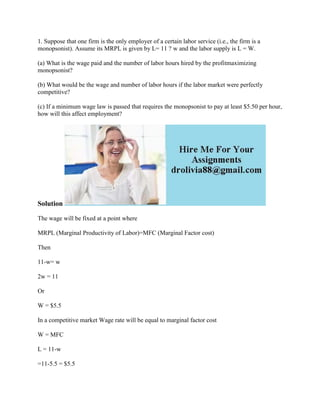Ce diaporama a bien été signalé.
Le téléchargement de votre SlideShare est en cours. ×

# 1- Suppose that one firm is the only employer of a certain labor servi.docx

Publicité
Publicité
Publicité
Publicité
Publicité
Publicité
Publicité
Publicité
Publicité
Publicité
PublicitéChargement dans…3
×

## Consultez-les par la suite

1 sur 2 Publicité

# 1- Suppose that one firm is the only employer of a certain labor servi.docx

1. Suppose that one firm is the only employer of a certain labor service (i.e., the firm is a monopsonist). Assume its MRPL is given by L= 11 ? w and the labor supply is L = W.
(a) What is the wage paid and the number of labor hours hired by the profitmaximizing monopsonist?
(b) What would be the wage and number of labor hours if the labor market were perfectly competitive?
(c) If a minimum wage law is passed that requires the monopsonist to pay at least \$5.50 per hour, how will this affect employment?
Solution
The wage will be fixed at a point where
MRPL (Marginal Productivity of Labor)=MFC (Marginal Factor cost)
Then
11-w= w
2w = 11
Or
W = \$5.5
In a competitive market Wage rate will be equal to marginal factor cost
W = MFC
L = 11-w
=11-5.5 = \$5.5
The wages are equal to the minimum wage, so there will not be any effect on employment and wages in the economy. The minimum wage is same as that of equilibrium wage corresponding to demand and supply of labor.
.

1. Suppose that one firm is the only employer of a certain labor service (i.e., the firm is a monopsonist). Assume its MRPL is given by L= 11 ? w and the labor supply is L = W.
(a) What is the wage paid and the number of labor hours hired by the profitmaximizing monopsonist?
(b) What would be the wage and number of labor hours if the labor market were perfectly competitive?
(c) If a minimum wage law is passed that requires the monopsonist to pay at least \$5.50 per hour, how will this affect employment?
Solution
The wage will be fixed at a point where
MRPL (Marginal Productivity of Labor)=MFC (Marginal Factor cost)
Then
11-w= w
2w = 11
Or
W = \$5.5
In a competitive market Wage rate will be equal to marginal factor cost
W = MFC
L = 11-w
=11-5.5 = \$5.5
The wages are equal to the minimum wage, so there will not be any effect on employment and wages in the economy. The minimum wage is same as that of equilibrium wage corresponding to demand and supply of labor.
.

Publicité
Publicité

### 1- Suppose that one firm is the only employer of a certain labor servi.docx

1. 1. 1. Suppose that one firm is the only employer of a certain labor service (i.e., the firm is a monopsonist). Assume its MRPL is given by L= 11 ? w and the labor supply is L = W. (a) What is the wage paid and the number of labor hours hired by the profitmaximizing monopsonist? (b) What would be the wage and number of labor hours if the labor market were perfectly competitive? (c) If a minimum wage law is passed that requires the monopsonist to pay at least \$5.50 per hour, how will this affect employment? Solution The wage will be fixed at a point where MRPL (Marginal Productivity of Labor)=MFC (Marginal Factor cost) Then 11-w= w 2w = 11 Or W = \$5.5 In a competitive market Wage rate will be equal to marginal factor cost W = MFC L = 11-w =11-5.5 = \$5.5
2. 2. The wages are equal to the minimum wage, so there will not be any effect on employment and wages in the economy. The minimum wage is same as that of equilibrium wage corresponding to demand and supply of labor.